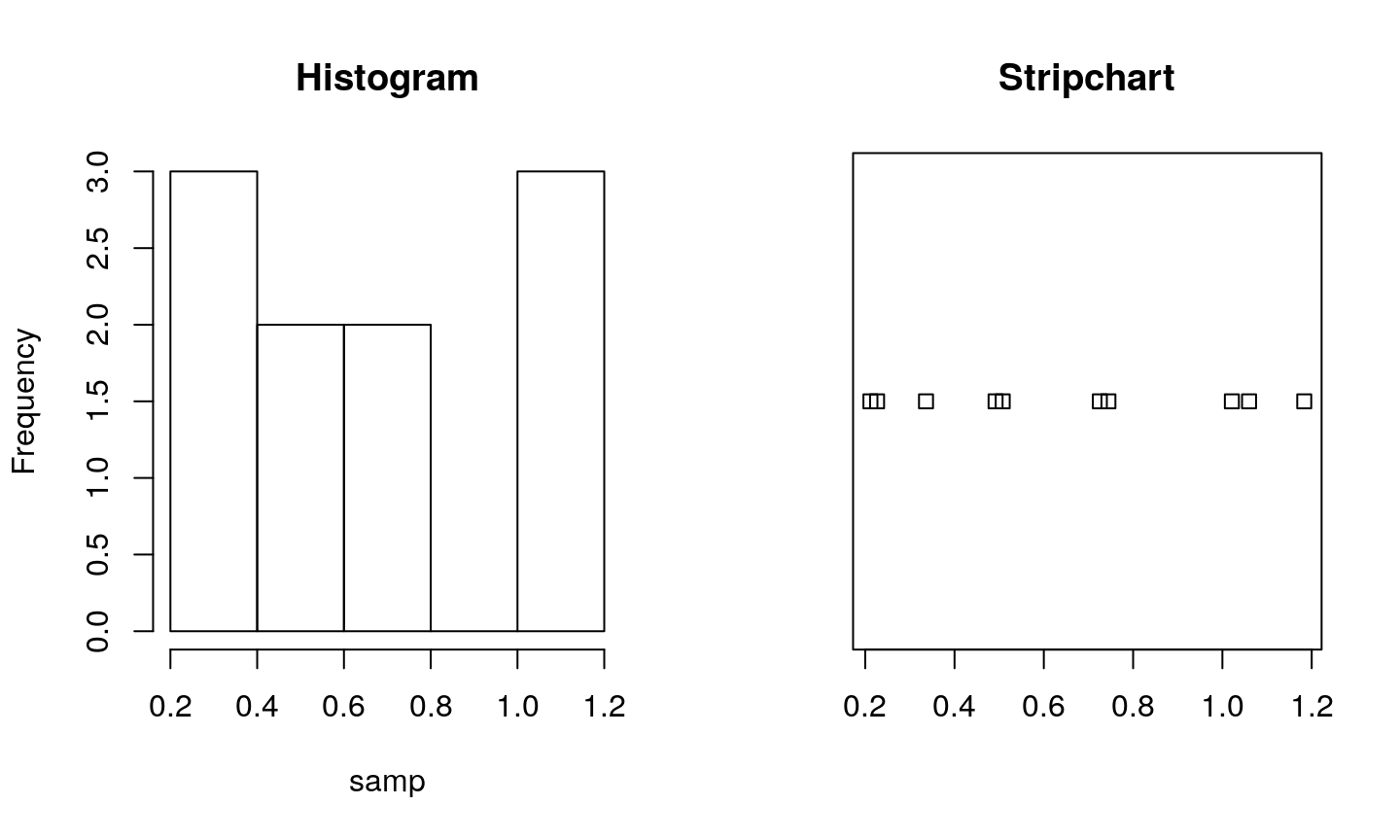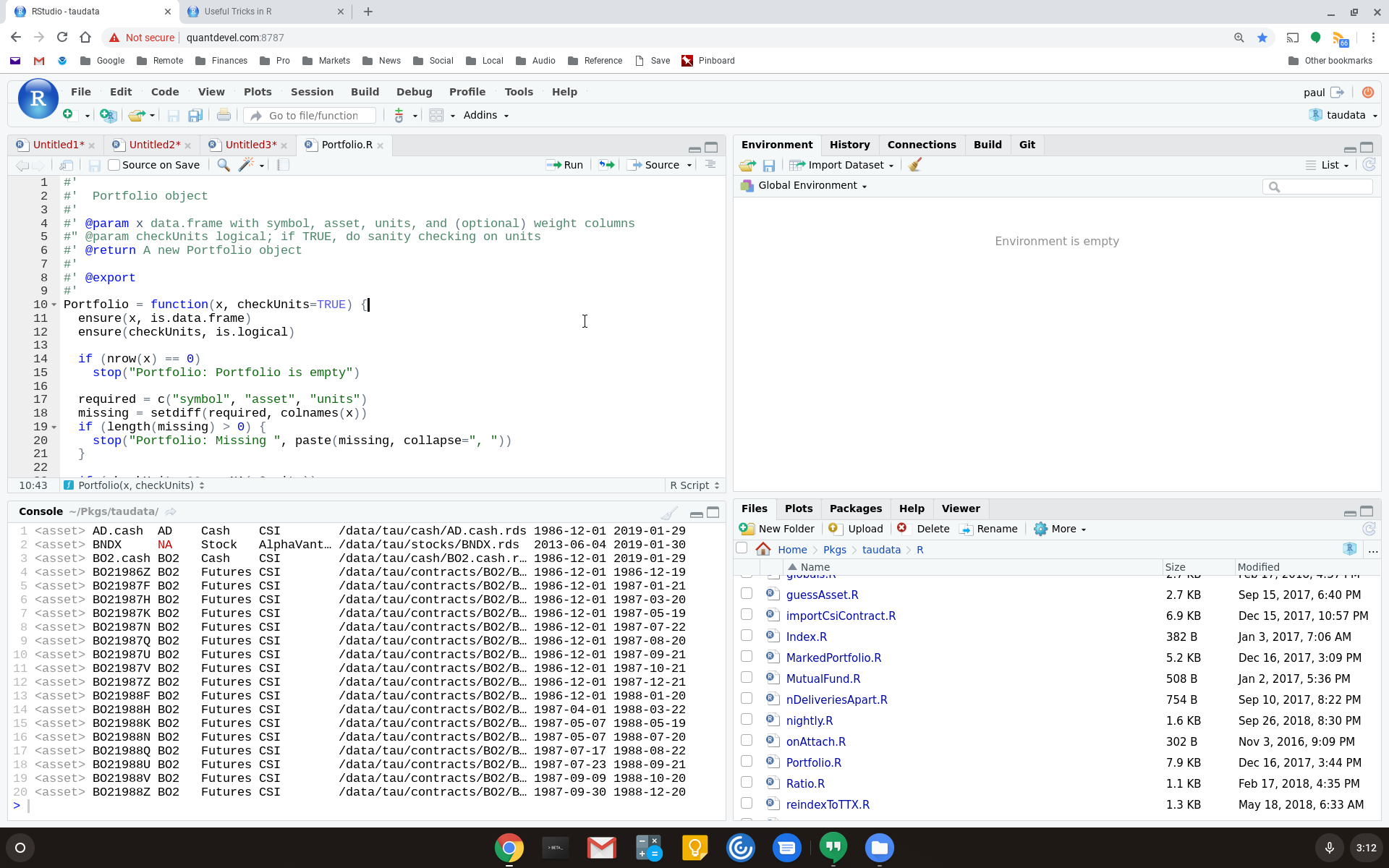February 2019

## .Last.value

The special variable `.Last.value` holds the value of the most recent expression at the command line.

```veryLongRunningFunction()   # Oops! Forgot to save result
x <- .Last.value            # Save it now```

Careful: `.Last.value` is overwritten with every new expression you type, so use it immediately!

## Right-hand assignment

```x <- 3     # Assign 3 to x
3 -> x     # Does the same thing

# Handy for capturing results
veryLongExpression +
anotherVeryLongExpression *
yetAnotherVeryLongExpression -> x

y %>% f
y %>% f %>% g
y %>% f %>% g %>% h -> x```

Very handy at the command line. Can’t recommend it for scripting: too odd.

## Double brackets extract exactly one element

Double brackets protect against accidentally selecting multiple elements.

```vec <- c(10, 20, 30, 40, 50)
vec[]    # 20
vec[[2:3]]  # Error: attempt to select more than one element
vec[]    # Error: subscript out of bounds

lst <- list(10, 20, 30, 40, 50)
lst[]    # 20
lst[[2:3]]  # Error: subscript out of bounds
lst[]    # Error: subscript out of bounds

lst[[i]]    # Error if 'i' does not select exactly one```

## Single brackets extract a subvector or sublist

```vec <- c(10, 20, 30, 40, 50)
vec      # c(20)
vec[2:3]    # c(20, 30)
vec      # NA

lst <- list(10, 20, 30, 40, 50)
lst      # list(20)
lst[2:3]    # list(20,30)
lst      # NULL

lst[i]      # Sublist? NULL? NA??```

## local(): Clean up intermediate values

These do the same thing:

```temp1 <- read_csv("hugeFile1.csv")
dframe <- inner_join(temp1, temp2, by = "name")
rm(temp1, temp2)

dframe <- local( {
inner_join(temp1, temp2, by = "name")
} )```
• `local()` returns value of last expression (here, the `inner_join`)
• Variables created within `local()` are discarded.

## Use `data_frame` instead of `data.frame`

### Quiz inspired by Hadley Wickham

The base function `data.frame` has some quirks.

```df <- data.frame(aardvark = "fred")
df\$a        # What value, if any, is this?

## Use `data_frame` instead of `data.frame`

### (continued)

The base function `data.frame` has some quirks.

```df <- data.frame(aardvark = "fred")
df\$a```
```##  fred
## Levels: fred```
`df\$b`
`## NULL`

Oops. Forgot `stringsAsFactors=FALSE`.

## Use `data_frame` instead of `data.frame`

### (continued)

The `dplyr` function `data_frame` is friendlier.

```library(dplyr)     # or library(tidyverse)

df <- data_frame(aardvark = "fred")
df\$aardvark        # "fred" (character, not factor)
df\$a               # NULL; Warning: Unknown or uninitialised column```

## Use `read_csv` instead of `read.csv`

`read_csv` is a replacement for `read.csv`.

```library(readr)     # or library(tidyverse)

• Faster than `read.csv` for big files
• Does not mangle column names (unlike `read.csv`)
• Does not convert strings to factors
• Nice progress bar for large files

## `str`: X-ray vision

Use `str` to quickly reveal data structure.

`str(airquality)`
```## 'data.frame':    153 obs. of  6 variables:
##  \$ Ozone  : int  41 36 12 18 NA 28 23 19 8 NA ...
##  \$ Solar.R: int  190 118 149 313 NA NA 299 99 19 194 ...
##  \$ Wind   : num  7.4 8 12.6 11.5 14.3 14.9 8.6 13.8 20.1 8.6 ...
##  \$ Temp   : int  67 72 74 62 56 66 65 59 61 69 ...
##  \$ Month  : int  5 5 5 5 5 5 5 5 5 5 ...
##  \$ Day    : int  1 2 3 4 5 6 7 8 9 10 ...```

## `zealot` will unpack lists and vectors

The zealot package provides an unpacking or destructuring operator, written `%<-%`, similar to Python or Matlab. These are identical:

```temp <- functionThatReturnsAList()
x <- temp[]
y <- temp[]
rm(temp)

library(zealot)
c(x, y) %<-% functionThatReturnsAList()```

Has many, many features. Check the help page.

## For small samples, use `stripchart`

Histograms of small samples aren’t useful. Use `stripchart` instead to get a sense of the data.

`stripchart(samp)`## Fit distributions with MASS::fitdistr

```samp <- rgamma(1000, 2, 3)
MASS::fitdistr(samp, "gamma")```
```##      shape       rate
##   2.1744783   3.3397465
##  (0.0907772) (0.1567427)```
• Very handy for quick, parametric Monte Carlo or bootstrap, for example
• Understands many distributions: beta, chi-squared, exponential, gamma, geometric, normal, Poisson, t, and many more
• See help page for full list of distributions

## Pull regression statistics from a linear model

Instead of printing the full `summary(m)` of a regression, you can pull individual regression statistics.

```m <- lm(dist ~ speed, data=cars)
`##  0.6438102`
`summary(m)\$coefficients`
```##               Estimate Std. Error   t value     Pr(>|t|)
## (Intercept) -17.579095  6.7584402 -2.601058 1.231882e-02
## speed         3.932409  0.4155128  9.463990 1.489836e-12```

## Pull regression statistics from a linear model

• `residuals`
• `coefficients`
• `sigma`
• `df` (degrees of freedom)
• `r.squared`
• `adj.r.squared`
• `fstatistic` (F statistic)
• `cov.unscaled` (Unscaled variance/covariance)

## End a pipeline with explicit `select`

What gets assigned to `x`?

```x <- dframe %>%
group_by(country) %>%
mutate(country_total = sum(population)) %>%
ungroup %>%
mutate(country_pct = revenue / country_total)```

## End a pipeline with explicit `select`

### (continued)

Ending with an explicit `select` makes the result obvious.

```x <- dframe %>%
group_by(country) %>%
mutate(country_total = sum(population)) %>%
ungroup %>%
mutate(country_pct = population / country_total) %>%
select(date, country, population, country_pct)```

## `with()` extracts individual columns

`with(dframe, expr)` means use columns of `dframe` to evaluation `expr`. For example, these are equivlent.

```cars\$speed * cars\$dist
with(cars, speed * dist)```

Handy for pulling out columns at end of pipline.

```# Want median diff. between 'after' and 'before' columns
full_join(df1, df2, by="name") %>%
with(median(after - before))```

## Tricks with RStudio panes

Suppose you’re working in RStudio . .## Tricks with RStudio: Zoom the editor

• Ctrl + Shift + 1
• Via menu: View -> Panes -> Zoom Source